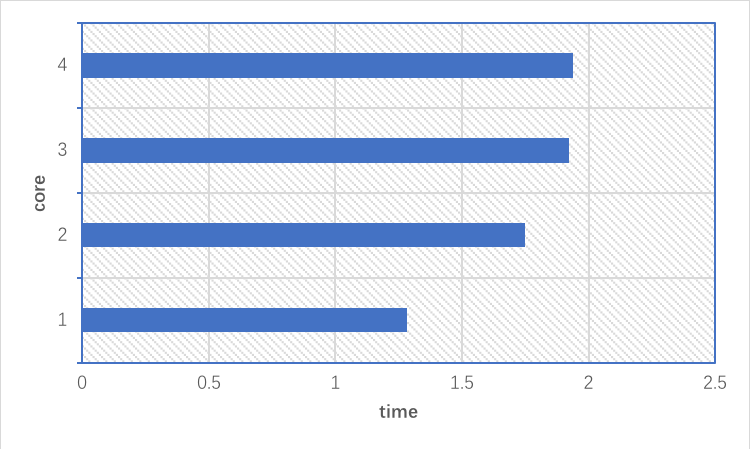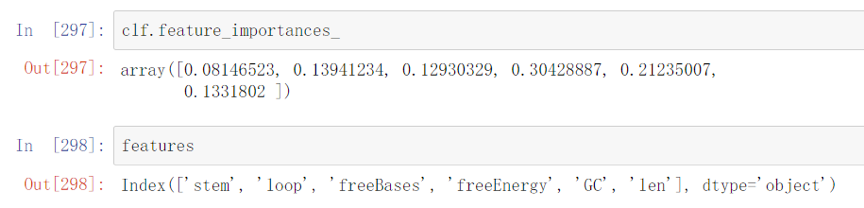# Team:Jilin China/Model/Screening System

RNA-based Thermosensors
Intelligent Screening System

• ## Introduction

During our repeated experiments, we found that the RNA-based thermosensors we designed were not all desirable. In order to speed up our experiments and reduce the probability of undesirable thermosensors, we developed an intelligent screening system for RNA-based thermosensors based on machine learning for use in subsequent experiments.

• ## Methodology

The RNA-based Thermosensor Intelligent Screening System is based on a random forest algorithm in machine learning, which is a perturb-and-combine technique specifically designed for trees. A diverse set of classifiers is created by introducing randomness in the classifier construction. The final prediction is decided by the average of each prediction of the individual classifiers.

### Basic concept

Random forest is an ensemble learning method for classification, regression and other tasks, that operate by constructing a multitude of decision trees at training time and outputting the class that is the mode of the classes (classification) or mean prediction (regression) of the individual trees. Random decision forests correct for decision trees' habit of overfitting to their training set.

Decision tree is a decision support tool that uses a tree-like graph or model of decisions and their possible consequences, including chance event outcomes, resource costs and utility.

The following parameters were used(Table.1):

Table 1. The parameters in the random forest

Parameter Value Type
n_estimators 1000 integer
criterion gini string
max_depth None None
min_samples_split 2 int
min_samples_leaf 1 int
min_weight_fraction_leaf 0. float
max_features sqrt(n_features) float
max_leaf_nodes None None
min_impurity_decrease 0. float
bootstrap True bool
oob_score True bool
n_jobs 1 int
random_state None None
verbose 0 int
warm_start False bool
class_weight None None

Firstly, we performed feature engineering on RNA sequences. Under the guidance of some papers, we can obtain many strong features: GC content, stem length, loop length, sequence length, number of free bases and free energy. Features of GC content and sequence length are easy to obtain, but we need to get RNA secondary structure before the features of number of free bases, stem length and loop length. Here, we used the principle of minimum free energy and dynamic programming algorithm to find the RNA secondary structure and its free energy.Figure 1. The features we extracted

As other classifiers, forest classifiers have to be fitted with two arrays: an array X of size [n_samples, n_features] holding the training samples, and an array Y of size [n_samples] holding the target class labels for the training samples.Here n_samples is our training samples and n_features is the number of features in the RNA-based thermosensors.

In random forests, each tree in the ensemble is built from a sample drawn with replacement (i.e., a bootstrap sample) from the training set. In addition, when splitting a node during the construction of the tree using the principle of the largest Gini coefficient, the split that is chosen is no longer the best split among all features. Instead, the split that is picked is the best split among a random subset of the features. As a result of this randomness, the bias of the forest usually slightly increases (with respect to the bias of a single non-random tree). However due to averaging, its variance also decreases, usually more than compensating for the increase in bias, hence yielding an overall better model.

In contrast to the original publication, our implementation combines classifiers by averaging their probabilistic prediction (i.e., Soft Voting), instead of letting each classifier vote for a single class.

The main parameters to adjust when using these methods is n_estimators and max_features. The former is the number of trees in the forest. For n_estimators, the larger is better, however it will take longer time to compute. In addition, it will not get significantly better after beyond a critical number of trees. For us, we use n_estimatores=1000 to train our model. The latter is the size of the random subsets of features to consider when splitting a node. The lower is greater for the reduction of variance, however the bias will increase. Empirical good default values are max_features=sqrt(n_features) for classification tasks, where n_features is the number of features in the RNA-based thermosensors. And when setting max depth of trees in our forest is None and the min samples split of training samples is 2 (i.e., when fully developing the trees),we get the best results. In addition, in random forests, we use bootstrapping samples and estimate the generalization accuracy on the out-of-bag(OOB) samples.Figure 2. Construction of the random forest

Finally, random forest also features the parallel construction of the trees and parallel computation of the predictions. However, instead of using all the cores of the computer, we used one core to build our random forest, which reduced inter-process communication overhead.Figure 3. The core we used and the time we needed

The relative rank of a feature used as a decision node in a tree can be used to assess the relative importance of that feature with respect to the predictability of the RNA-based thermosensor. Features used at the top of the tree contribute to the final prediction decision of a larger fraction of the input samples. The expected fraction of the samples they contribute to can thus be used as an estimate of the relative importance of the features. Here, the fraction of samples a feature contributes to is combined with the decrease in impurity from splitting them to create a normalized estimate of the predictive power of that feature.Figure 4. Feature importance evaluation

• ## Results

In this work, we increased the probability of getting a desirable RNA-based thermosensor from 47% to about 65%, and determined GC content and free energy as the most important features of RNA-based thermosensors. The two features are very important and useful for the our following design of RNA-based thermosensors, and reduced workload and increased work efficiency.

• ## References

•  Breiman L. Arcing classifier (with discussion and a rejoinder by the author)[J]. The annals of statistics, 1998, 26(3): 801-849.
•  Ho T K. Random decision forests[C]//Document analysis and recognition, 1995., proceedings of the third international conference on. IEEE, 1995, 1: 278-282.
•  Barandiaran I. The random subspace method for constructing decision forests[J]. IEEE transactions on pattern analysis and machine intelligence, 1998, 20(8).
•  Trevor H, Robert T, JH F. The elements of statistical learning: data mining, inference, and prediction[J]. 2009.
•  Zheng L L, Qu L H. Computational RNomics: Structure identification and functional prediction of non-coding RNAs in silico[J]. Science China Life Sciences, 2010, 53(5): 548-562.
•  Wuchty S, Fontana W, Hofacker I L, et al. Complete suboptimal folding of RNA and the stability of secondary structures[J]. Biopolymers: Original Research on Biomolecules, 1999, 49(2): 145-165.
•  Mathews D H, Sabina J, Zuker M, et al. Expanded sequence dependence of thermodynamic parameters improves prediction of RNA secondary structure1[J]. Journal of molecular biology, 1999, 288(5): 911-940.
•  Breiman L. Random forests[J]. Machine learning, 2001, 45(1): 5-32.
•  Wolpert D H, Macready W G. An efficient method to estimate bagging's generalization error[J]. Machine Learning, 1999, 35(1): 41-55.